# What are the Types of Pointers in C++ with Examples?Do you know what is the purpose of Pointers in C++? C++ is a powerful and widely used programming language and many concepts in C++ are important for you to understand, Pointers in C++ is one of them. Let us try to understand this topic by knowing their types along with some examples.

Still, if you have any questions related to Pointers or any issues while solving your C++ assignment then you can get C++ homework help by hiring the proficient experts at CodingZap.

But before we start gathering knowledge about the pointers in C++, let us try to find out the purpose & importance of pointers in C++. This will help to clarify what are pointers in CPP & why to use pointers in CPP.

## What Are Pointers In CPP? Get To Know

Any variable in the programming languages is stored in the memory. Inside the memory, there are some spaces with proper addresses. Like houses have unique addresses. Similarly, the variables that are stored in the computer memory have different addresses. Using these addresses, we can differentiate them.

Pointers store these addresses inside of them. pointers act as a variable. This means they will occupy some position inside the memory. So, every pointer must have its address. Along with that, the pointers can able to store the address of another variable inside of it.

General Syntax: data-type *pointer-name;

Along with that, with a pointer, there is an asterisk (*) sign present. This asterisk sign acts as the ticket to enter the address of the variables. If a pointer has an asterisk with it, it will show the value of the variable. The address of the variable should be provided there. This is a special function.

Different data types have different kinds of pointers. Also, the data type size plays an important role in marking the address of the pointers. Like for any pointer having the type int has the size 4 byte. The following is an examples of pointers:

```				```

int    *zap; // Integer Pointer
float  *coding; // Float Pointer
char   *one; // Character Pointer

```
```

## How To Use Pointers In CPP?

Now, it is time to understand the declaration process of the C++ pointers. Many students made mistakes while writing or working on a piece of code where the pointers are necessary to use. Follow the below steps one by one to define it correctly:

1. First, write down the name of the pointer without using any sign.
2. Now, use the variable name along with the unary operator (&) after the assignment signature.
3. Now, paste the data type of the variable before the pointer name to make it correct.
4. If you want to access the value of the variable, then use the asterisk sign (*) before the pointer name.

Follow this pattern whenever you are working with C++ pointers. This practice should be done in your beginner’s days. After becoming a pro-coder, you can leave this practice.

## Why Use Pointers In Cpp? Read Below

Pointers are not only used in C++. But this is also used in various programming languages. This helps to reduce the space complexity of the programming. Along with that, it helps to reduce the problems related to function.

There are few reasons or fields present where the need to use C++ pointers becomes inevitable. They are the following:

Reason 1:

If any variable is thrown to another function as an argument, then sometimes there might be some issues. Sometimes the value of the variable gets changed unnecessarily. Using the pointers, we can able to do anything with the variable. But the value will not change.

Reason 2:

Sometimes, there is a need to use dynamic memory allocation. This is the special feature. This is completely based on the pointers in C++. Also, sharing one array of information with other functions can easily be done with the help of pointers. There just we need to provide the starting index address.

For those reasons, pointers are highly used. In every field, the pointers are included in the programs for better structure of the program. Pointers help to think about the address of the variables. Their allocation process can be understood using pointers. This means the advantage of using pointers is infinite.

Also, if you wish to know more about C++ and its uses then you can read our article to have a more clear understanding.

## How Pointers Work In C++?

Before going to understand the working process of C++ pointers, we need to understand the assigning process of a variable in any programming language. When a variable is declared it is stored in the memory with some address. Inside the memory address, the value gets stored.

Now, a pointer declared with the same data type can access the variable, if it gets its address. Now, to give it the address of the variable, the unary operator (&) should be used along with the variable name. So, it is now pointing to the address of the variable.

Still, the pointer not getting the value of the variable. To provide the value, we have to write the asterisk sign (*) before the pointer name. Now, the pointer can enter into the memory address & do all the necessary operations on the value itself.## Why Use Pointers In Cpp? Read Below

Pointers are not only used in C++. But this is also used in various programming languages. This helps to reduce the space complexity of the programming. Along with that, it helps to reduce the problems related to function.

There are few reasons or fields present where the need to use C++ pointers becomes inevitable. They are the following:

Reason 1:

If any variable is thrown to another function as an argument, then sometimes there might be some issues. Sometimes the value of the variable gets changed unnecessarily. Using the pointers, we can able to do anything with the variable. But the value will not change.

Reason 2:

Sometimes, there is a need to use dynamic memory allocation. This is the special feature. This is completely based on the pointers in C++. Also, sharing one array of information with other functions can easily be done with the help of pointers. There just we need to provide the starting index address.

For those reasons, pointers are highly used. In every field, the pointers are included in the programs for better structure of the program. Pointers help to think about the address of the variables. Their allocation process can be understood using pointers. This means the advantage of using pointers is infinite.

Also, if you wish to know more about C++ and its uses then you can read our article to have a more clear understanding.

## What Are The Types Of Pointers In C++?

The types of pointers are not so important. There is only one type of pointer mainly used on a large scale. That is the normal pointer. Along with that, there are two other types of pointers in C++. These things need to be discussed in a better manner.

But let us first make a list of the possible types of pointers in C++.

1. Normal Pointer
2. Void Pointer
3. Null Pointer

Let us try to know more about them one by one briefly.

## How To Implement Pointers In CPP?

Now, it is time to understand the implementation process of the pointers in the C++ programming language. The above-discussed pointers are classified as the pointers that are normally used by a programmer. They can be classified as beginner-level pointers.

Later, we will discuss some advanced operations with pointers. So, let us start our discussion!

• ### Normal Pointers In C++

By the name of the pointer, we can assume that it is going to be the most simple pointer declaration process. It is the foundation on which different operations of Pointer are executed. The normal & simple syntax of the pointer will be used here.

Syntax: data-type *pointer-name;

Apart from defining pointers in C++, you might have learned to declare pointers in C programming language but still, if you have no clue how to do that then we would suggest you get Expert C help from the best developers.

Now, let’s learn about declaring the normal Pointers in C++ with the help of example coding.

Code To Define The Normal Pointer In C++ Language:

```				```
#include <iostream> using namespace std;
int main() {
int  zap = 5; // Variable Declaration
int  *one; // Pointer Declaration
one = &zap; // Pointer To Variable
cout << "Address Value : "<< one<< endl;
cout << "Value : "<< *one << endl; return 0}

```
```
##### Steps Of The Program:
1. At first, a variable is declared with the same values.
2. Now, a pointer will be initiated with the same data type as the variable.
3. Now, using the unary operator (&) the memory address of the variable will be stored to the pointer.
4. We will print the address of the variable & using the asterisk sign (*) the value will also be printed.

Let us try to find out the output of the above code. This will help to understand the normal pointers in C++.

Output: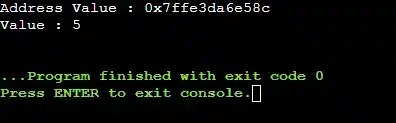From the above output, we can see the first data is the address of the variable. After printing the memory address, it prints the value of the variable using the pointer declared in the program.

• ### Void Pointers In C++

Void pointers are those pointers, that can be used in every data type. This means these pointers are declared in void form. Then, as per our need, we can type case them to any other data type. This helps to reduce the pointer usability in the program. Only one pointer can be used in different cases.

This pointer is highly used by programmers who are unaware of the variable datatype. To bypass the compilation error, the void pointer is used that can be assigned to any variable.

Syntax: void *pointer-name;

Code To Define The Void Pointer In C++ Language:

```				```
#include <iostream>using namespace std;
int main(){
int zap = 5; // Variable Declaration
void *one; // Void Pointer Declaration
one = &zap; // Pointer To Variable
cout<<"Integer Value: << *((int*)one)) // Type Casting Pointer
return 0;}

```
```
##### Steps Of The Program:
1. At first, a variable is declared with the value.
2. Now, a void pointer will be declared. Hence, no data type should be provided.
3. Now, using the unary operator (&) the assignment of the variable will be done.
4. Now, as the value is printed in the program, the type-casting should be done. Here, the value is the integer one, so the pointer should be type-casted to the int format.

Let us try to find out the output of the above code. This will help to understand the void pointers in C++.

Output: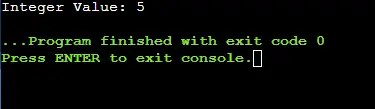The above output clearly shows that a void pointer can store the memory location after performing the assignment operation. The integer data came as the output as the type-casting performed in a good manner.

• ### Null Pointers In C++

This is another type of pointer. In this case, a pointer is declared. But it will have the initial value as Null. This means a later value is going to be provided there. This is also a pre-step of the normal pointers. Sometimes a normal pointer is declared as a Null pointer. After that, the value will be provided there.

Code To Demonstrate The Declaration Of Null Pointers:

```				```
#include <iostream>using namespace std;
int main(){
int *zapone = NULL; // Null Pointer Declaration
cout << "Value Of Pointer: " << zapone;
return 0;}

```
```
##### Steps Of The Program:
1. A pointer will be declared with the asterisk sign (*) along with a data type in the program.
2. Now, a null value will be provided to initialize it completely. It can now be ready to hold any similar data type value in the future.
3. We can also print the pointer to check the implementation correctness.

Let us try to find out the output of the above code. This will help to understand the null pointers in C++.

Output: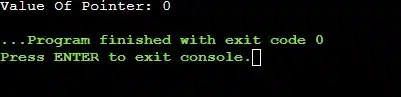The above output clearly shows that the Null Pointer is declared correctly. It can now hold memory addresses of any similar data types. As the pointer is not pointing to any memory location, it is giving the zero value as output.

Now, let us move to understand some of the advanced operations on the C++ pointer variable that need to be used in many programming problems. Let us know all of them one by one briefly.

• ### Double Pointer In CPP

Double pointer is termed as the Pointer To Pointer operation in C++. Many experts call it “Pointers Pointers”. The simple definition is a pointer is pointing towards another pointer that is again pointed to a variable.

General Syntax: data-type **pointer-name;

Here, the first pointer is used to store the address of the variable. The second pointer is used to store the address of the first pointer that is having the address of the variable. So, indirectly the second pointer will also focus on the variable address.

Code To Define The Declaration Of Double Pointer In CPP:

```				```

#include <iostream>
using namespace std;
int main()
{
int  coding = 2023; // Variable Declaration
int  *zap; // First Pointer Declaration
int  **one; // Double Pointer Declaration

zap = &coding; // Pointer To Variable
one = &zap; // Pointer To Pointer

cout << "Value Of Varible: "<< coding << endl;
cout << "Value Of First Pointer 'Zap': "<< *zap << endl;
cout << "Value Of Double Pointer 'One' : "<< **one << endl;
return 0;

}

```
```
##### Steps Of The Program:
1. At first, a variable will be declared along with the value.
2. Now, a first pointer with one asterisk sign (*) and a double pointer with two asterisk signs (*) will be initialized. Remember, all of them will have the same data type with variables.
3. Now, the first pointer name will get the variable address. The double-pointer will get the address of the first pointer.
4. Now, we will print the same variable values using the pointer variables.

Output:The output clearly shows that the function pointer works properly. The task of the function was to add two integers. The pointer that is pointed to the function can do the work. The output data is printed on the window.

• ### File Pointer In CPP

The file pointer is not a simple kind of pointer that can be used in any program based on the need. The File is a special operation in programming languages that can be performed with the help of Data Structures. The pointers that are used there are known as the file pointer.

In simple terms, when there is a term present “File” before the declaration of any pointer, it becomes the file pointer. Here, the File will be the data type of the pointer that can be used for file operations like opening any file or reading any file, etc.

General Syntax: File *pointer-name;

Code To Demonstrate The Use Of File Pointer In CPP:

```				```

#include <iostream>
using namespace std;
int main()
{
FILE *zap;
zap = fopen("zapone.txt", "w"); // File Pointer Open
fclose(zap); // File Pointer Close
return 0;

}

```
```
##### Steps Of The Program:
1. A file pointer will be declared in the program using the File data type.
2. Now, the fopen() function will be used to open a new file in the write mode. The pointer will be used in this statement.
3. Now, it is a good practice to close a file after opening. So, the fclose() will be used along with the pointer name.

Output: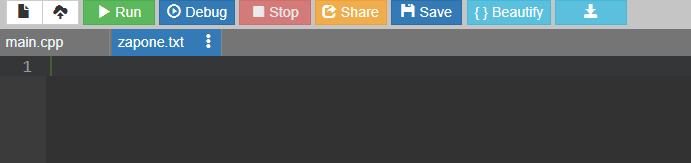The above output shows that a new file zapone.txt is opened in the IDE. As we have to perform any write operation, the file is completely blank. You will not get any output in the window as nothing printing operation is performed.

• ### Function Pointers In CPP

Here, the function will be used as the pointer in the program. Students often misunderstand the topic by sending pointers to the function. In Pointer To Function, one or more pointers are shared to one function, but here one function becomes a pointer.

In the case of the function pointers, one important thing is to put all the arguments of the function during the declaration process. There is no need to use any data types. Also. The pointer can only be assigned to another function.

General Syntax: return data-type of function (*pointer-name)(argument 1, argument 2…);

Code To Demonstrate The Declaration Of A Function Pointer:

```				```

#include <iostream>
using namespace std;
{
return a + b; // Addition Of Values
}
int main()
{
int (*zap)(int, int); // Function Pointers
int res = zap(4, 2); // Calling The Function
cout << "The Value Is: " << res << endl;
return 0;
}

```
```
##### Steps Of The Program:
1. At first, one user-defined function will be declared that will perform certain operations.
2. Now, in the main function, the function pointer will be declared by following the syntax.
3. Now, assign the function name to the pointer.
4. Provide the argument values to the pointer & if it returns something store it in a variable.
5. Now, print the result in the program.

Output: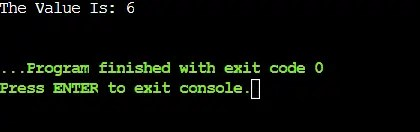The output clearly shows that the function pointer works properly. The task of the function was to add two integers. The pointer that is pointed to the function can do the work. The output data is printed on the window.

• ### Array Pointer In CPP

Array pointers are the C++ pointers that are associated with the array concept. The concept says that if a pointer can be pointed to the first element address, then using the address we can get other elements of the array.

In an array, every element is stored in the continuous memory addresses. If the first element is stored in memory location 2000, then the second element will be stored in 2001 memory location, and so on. Using this concept, the Array Pointer or Pointer To Array can be determined.

General Syntax: Pointer-Name = Array-Name;

Code To Demonstrate The Declaration Of An Array Pointer:

```				```

#include <iostream>
using namespace std;
int main()
{
int coding = {10, 20, 30, 40, 50}; // Array Name
int *zap; // Integer Pointer

zap = coding; // Pointer To Array Concept

cout << "The First Element: " << *coding << endl;
cout << "The Second Element: " << *(coding+1) << endl;
cout << "The Third Element: " << *(coding+2) << endl;
cout << "The Fourth Element: " << *(coding+3) << endl;
cout << "The Fifth Element: " << *(coding+4) << endl;
return 0;
}

```
```
##### Steps Of The Program:
1. At first, an array with some values should be declared.
2. Now, a pointer will be declared with the same data type as the array. Now, the pointer will be assigned to the array name.
3. We can now print the array elements with the pointer along with an asterisk sign (*). If we add 1 number with the pointer, it will point to the second element of the array.

Output: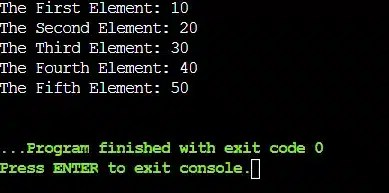In the output screenshot, we can see that each & every element of the array is printed correctly. So, the pointer that was taking the first address of the array works completely well. So, it is another way to print all the elements of an array.

• ### Pointer To Function In C++

We have already understood the Function Pointer in C++, it is time to understand the Pointer To Function process and get the differences between them. In simple terms, the Pointer To Function is used to share the address of any variable to another function using pointers.

Suppose, you have some confidential data in one function & you are bound not to change that variable in that same function. In such a case, you need to share the pointer to some other function. This can be seen a lot in competitive exams where a main function is already declared using a pointer to the function process.

Code To Define The Declaration Of Pointer To Function In CPP:

```				```

#include <iostream>
using namespace std;
void print(int *zap, int *one) // Function Accepting Pointers
{
cout << "The First Value Is: " << *zap << endl;
cout << "The Second Value Is: " << *one << endl;
}
int main()
{
int a = 10, b= 20; // Some Declared Variables
print (&a, &b); // Calling Function With Addresses
return 0;
}

```
```
##### Steps Of The Program:
1. In the main function, some variables are declared with some values.
2. Now, a user-defined function is called with some arguments. Here, the arguments will be the address of the variables.
3. In the user-defined function, to catch the address arguments, some pointers need to be used.
4. Using those pointers, the value can be printed as we have performed similar tasks previously.

Output: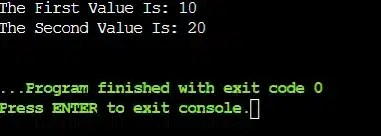From the above output, we can see that the values are successfully printed in another function. But, we have not shared the variable directly. Rather, we used the pointer techniques to catch the values from the main function & print it in the program.

## Conclusion:

As we saw, pointers in C++ are a very important topic.

We need to always remember what are pointers in CPP. Also, we need to gain more knowledge about why to use pointers in CPP by doing more & more practice.

Still having issues understanding, It is advisable to clear the basics of the C++ programming language, you can always hire a programming tutor from CodingZap. It will be easy for us to gain knowledge about this topic in an easy & proper manner.

So, hope you have liked this piece of article. Share your thoughts in the comments section and let us know if we can improve more.